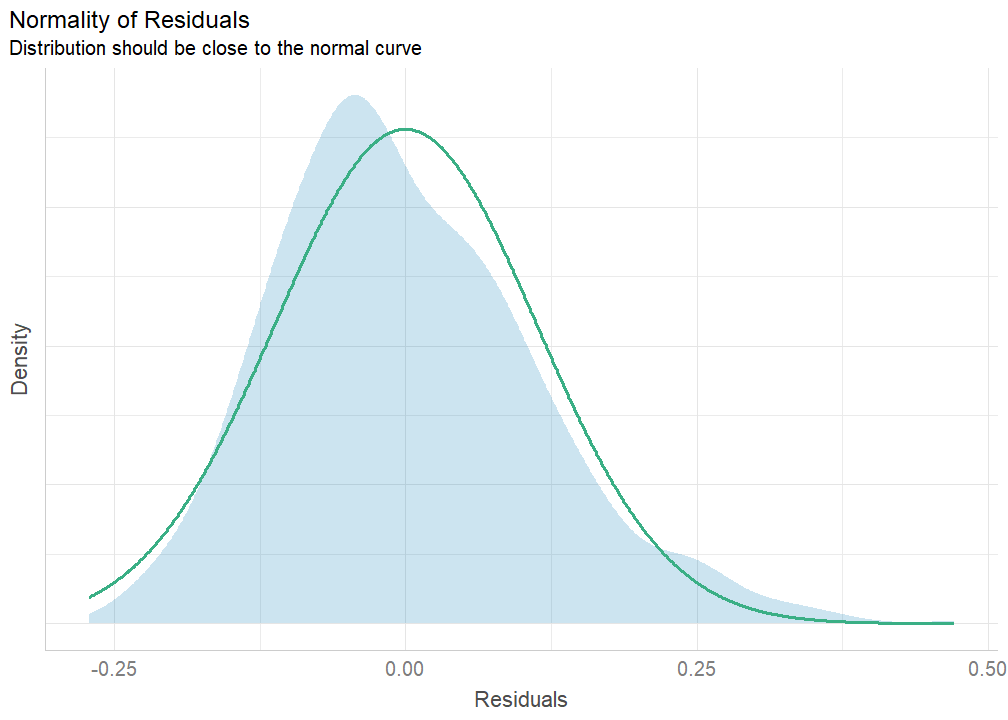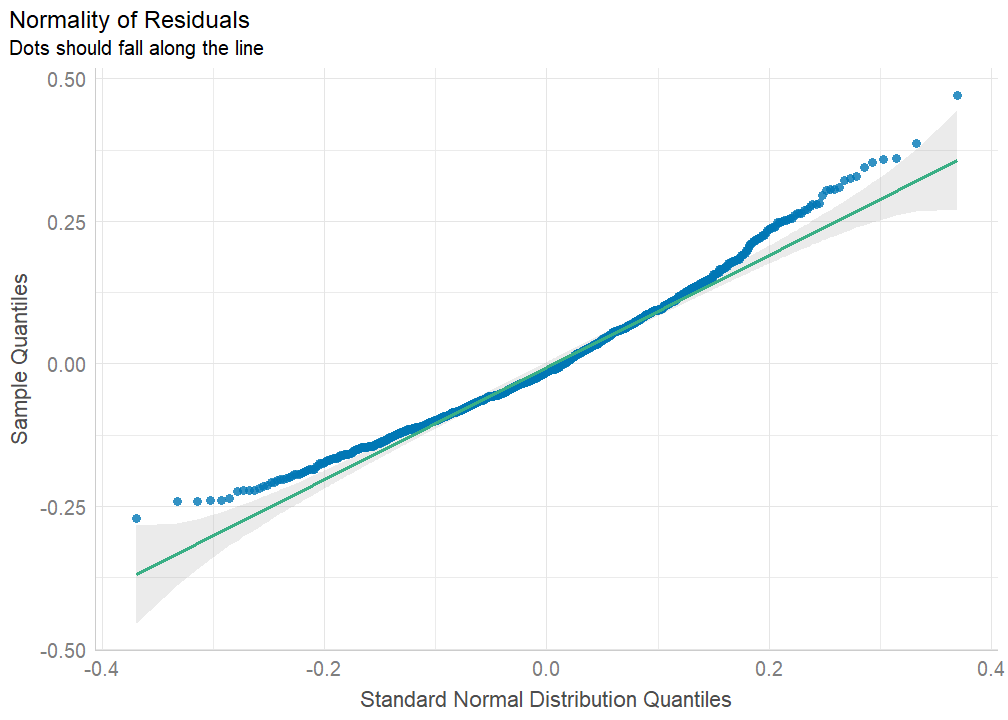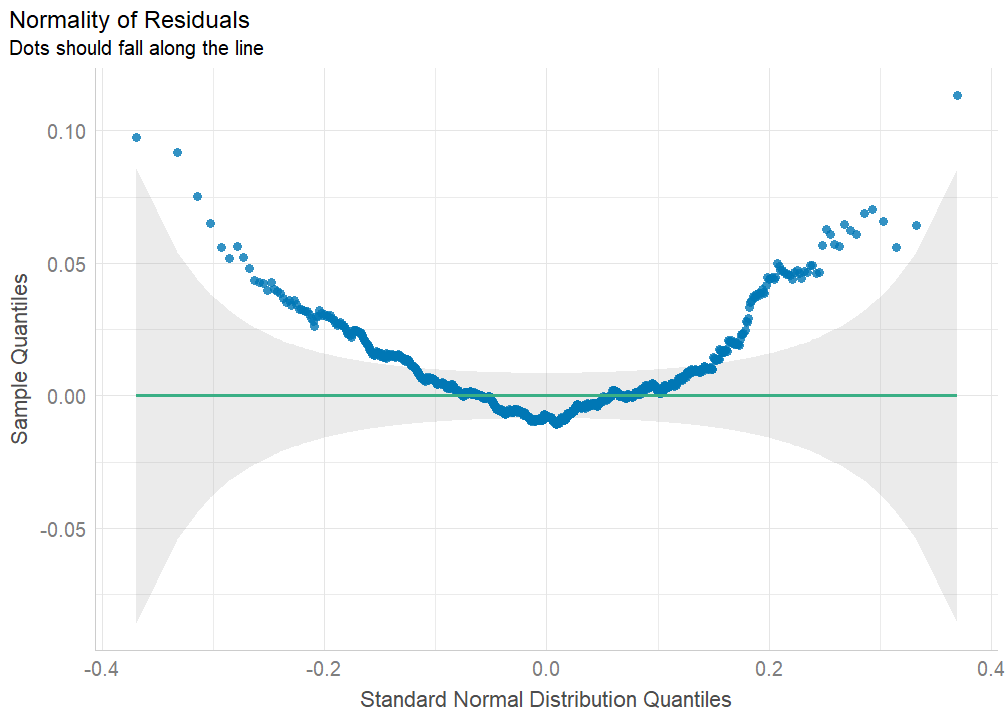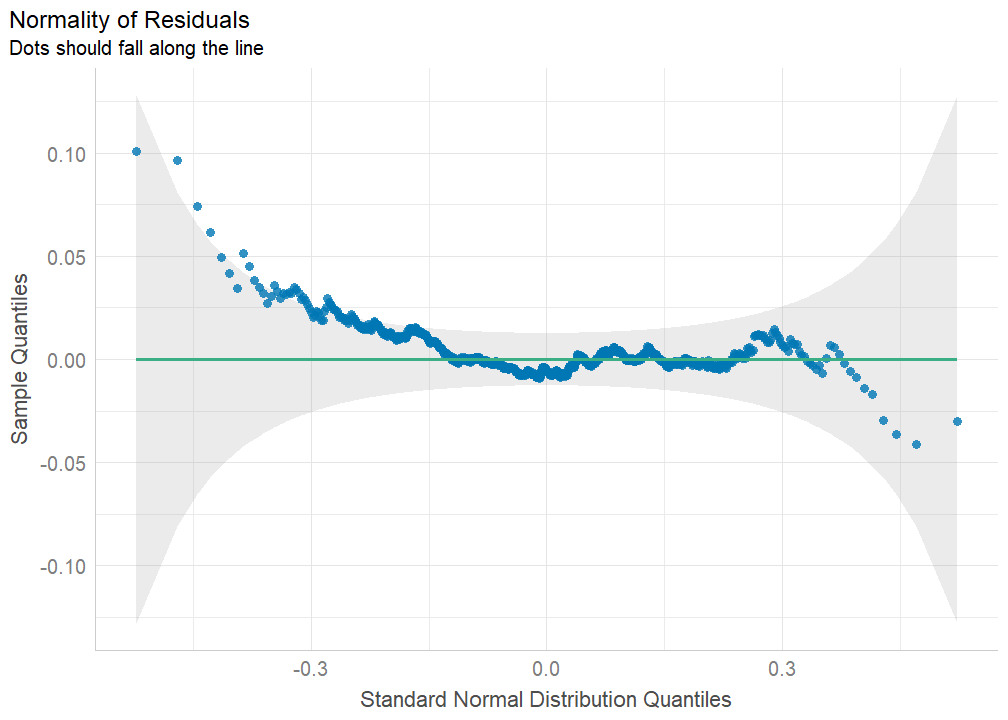# Testing the Assumptions of ANOVAs

## Foreword by Henrik Singmann

As all statistical models, ANOVAs have a number of assumptions that should hold for valid inferences. These assumptions are:

1. Observations are i.i.d.: i.i.d. stands for “independent and identically distributed”. Independent means that, once the model is specified, the conditional observations (i.e., residuals) are independent of each other (i.e., knowing the value of one residual does not allow you to infer the value of any other residual). Identically distributed means that all observations are generated by the same data-generating process.
2. Homogeneity of Variances: the variances across all the groups (cells) of between-subject effects are the same.
3. Sphericity: For within-subjects effects, sphericity is the condition where the variances of the differences between all possible pairs of within-subject conditions (i.e., levels of the independent variable) are equal. This can be thought of as a within-subjects’ version of the Homogeneity of Variances assumption.
4. Normality of residuals: The errors used for the estimation of the error term(s) (MSE) are normally distributed.

The most important assumption generally is the i.i.d. assumption (i.e., if it does not hold, the inferences are likely invalid), specifically the independent part. This assumption cannot be tested empirically but needs to hold on conceptual or logical grounds. For example, in an ideal completely between-subjects design each observation comes from a different participant that is randomly sampled from a population so we know that all observations are independent. Often, we collect multiple observations from the same participant in a within-subject or repeated-measures design. To ensure the i.i.d. assumption holds in this case, we need to specify an ANOVA with within-subject factors. However, if we have a data set with multiple sources of non-independence – such as participants and items – ANOVA models cannot be used but we have to use a mixed model.

The other assumptions can be tested empirically, either graphically or using statistical assumption tests. However, there are different opinions on how useful statistical assumptions tests are when done in an automatic manner for each ANOVA. Whereas this is the position taken in some statistics books, this runs the risk of reducing the statistical analysis to a “cookbook” or “flowchart”. Real life data analysis is often more complex than such simple rules. Therefore, it is often more productive to explore ones data using both descriptive statistics and graphical displays. This data exploration should allow one to judge whether the other ANOVA assumptions hold to a sufficient degree. For example, plotting ones ANOVA results using afex_plot and including a reasonable display of the individual data points often allows one to judge both the homogeneity of variance and the normality of the residuals assumption.

Let us take a look at all three empirically testable assumptions in detail. ANOVAs are often robust to light violations to the homogeneity of variances assumption. If this assumption is clearly violated, we have learned something important about the data, namely variance heterogeneity, that requires further study. Some further statistical solutions are discussed below.

If the main goal of an ANOVA is to see whether or not certain effects are significant, then the assumption of normality of the residuals is only required for small samples, thanks to the central limit theorem. As shown by Lumley et al. (2002), with sample sizes of a few hundred participants even extreme violations of the normality assumptions are unproblematic. So mild violations of this assumptions are usually no problem with sample sizes exceeding 30.

Finally, the default afex behaviour is to correct for violations of sphericity using the Greenhouse-Geisser correction. Whereas this default may in some situation produce a small loss in statistical power, this seems preferable to a situation in which violations of sphericity are overlooked and tests become anti-conservative (i.e., more false positive results).

Thus, my position as the afex developer is that an appropriate exploratory data analysis is often better than just blindly applying statistical assumption tests. Nevertheless, assumption tests are of course an important tool in the statistical toolbox and can be helpful in many situations. Thus, I am thankful to Mattan S. Ben-Shachar who has provided them for ANOVAs in afex. The following text provides his introduction to the assumption tests based on the performance and see packages.

## Testing the Empirically Testable Assumptions

afex comes with a set of built-in functions to help in the testing of the assumptions of ANOVA design. Generally speaking, the testable assumptions of ANOVA are1:

1. Homogeneity of Variances: the variances across all the groups (cells) of between-subject effects are the same. This can be tested with performance::check_homogeneity().
2. Sphericity: For within-subjects effects, sphericity is the condition where the variances of the differences between all possible pairs of within-subject conditions (i.e., levels of the independent variable) are equal. This can be thought of as a within-subjects’ version of the Homogeneity of Variances assumption, and can be tested with performance::check_sphericity().
3. Normality of residuals: The errors used for the estimation of the error term(s) (MSE) are normally distributed. This can be inferred using performance::check_normality().

What follows is a brief review of these assumptions and their tests.

library(afex)
library(performance) # for assumption checks

## Homogeneity of Variances

This assumption, for between subject-designs, states that the within group errors all share a common variance around the group’s mean. This can be tested with Levene’s test:

data(obk.long, package = "afex")

o1 <- aov_ez("id", "value", obk.long,
between = c("treatment", "gender"))
## Warning: More than one observation per design cell, aggregating data using fun_aggregate = mean.
## To turn off this warning, pass fun_aggregate = mean explicitly.
check_homogeneity(o1)
## OK: There is not clear evidence for different variances across groups (Levene's Test, p = 0.350).

These results indicate that homogeneity is not significantly violated.

### What to do when assumption is violated?

ANOVAs are generally robust to “light” heteroscedasticity, but there are various other methods (not available in afex) for getting robust error estimates.

Another alternative is to ditch this assumption altogether and use permutation tests (e.g. with permuco) or bootstrapped estimates (e.g. with boot).

## Sphericity

data("fhch2010", package = "afex")

a1 <- aov_ez("id", "log_rt", fhch2010,
within = c("density", "frequency", "length", "stimulus"))
## Warning: More than one observation per design cell, aggregating data using fun_aggregate = mean.
## To turn off this warning, pass fun_aggregate = mean explicitly.

We can use check_sphericity() to run Mauchly’s test of sphericity:

check_sphericity(a1)
## Warning in summary.Anova.mlm(object\$Anova, multivariate = FALSE): HF eps > 1 treated as 1
## Warning: Sphericity violated for:
##  - length:stimulus (p = 0.021)
##  - task:length:stimulus (p = 0.021).

We can see that both the error terms of the length:stimulus and task:length:stimulus interactions significantly violate the assumption of sphericity at p = 0.021. Note that as task is a between-subjects factor, both these interaction terms share the same error term!

### What to do when assumption is violated?

• For ANOVA tables, a correction to the degrees of freedom can be used - afex offers both the Greenhouse-Geisser (which is used by default) and the Hyunh-Feldt corrections.
• For follow-up contrasts with emmeans, a multivariate model can be used, which does not assume sphericity (this is used by default since afex 1.0).

Both can be set globally with:

afex_options(
correction_aov = "GG", # or "HF"
emmeans_model = "multivariate"
)

## Normalicy of Residuals

The normalicy of residuals assumption is concerned with the errors that make up the various error terms in the ANOVA. Although the Shapiro-Wilk test can be used to test for deviation from a normal distribution, this test tends to have high type-I error rates. Instead, one can visually inspect the residuals using quantile-quantile plots (AKA qq-plots). For example:

data("stroop", package = "afex")

stroop1 <- subset(stroop, study == 1)
stroop1 <- na.omit(stroop1)

s1 <- aov_ez("pno", "rt", stroop1,
within = c("condition", "congruency"))
## Warning: More than one observation per design cell, aggregating data using fun_aggregate = mean.
## To turn off this warning, pass fun_aggregate = mean explicitly.
is_norm <- check_normality(s1)

plot(is_norm)plot(is_norm, type = "qq")If the residuals were normally distributed, we would see them falling close to the diagonal line, inside the 95% confidence bands around the qq-line.

We can further de-trend the plot, and show not the expected quantile, but the deviation from the expected quantile, which may help reducing visual bias.

plot(is_norm, type = "qq", detrend = TRUE)Wow! The deviation from normalicy is now visually much more pronounced!

### What to do when assumption is violated?

As with the assumption of homogeneity of variances, we can resort to using permutation tests for ANOVA tables and bootstrap estimates / contrasts.

Another popular solution is to apply a monotonic transformation to the dependent variable. This should not be done lightly, as it changes the interpretability of the results (from the observed scale to the transformed scale). Luckily for us, it is common to log transform reaction times, which we can easily do2:

s2 <- aov_ez("pno", "rt", stroop1,
transformation = "log",
within = c("condition", "congruency"))
## Warning: More than one observation per design cell, aggregating data using fun_aggregate = mean.
## To turn off this warning, pass fun_aggregate = mean explicitly.
is_norm <- check_normality(s2)

plot(is_norm, type = "qq", detrend = TRUE)Success - after the transformation, the residuals (on the log scale) do not deviate more than expected from errors sampled from a normal distribution (are mostly contained in the 95%CI bands)!

1. There is also the assumptions that (a) the model is correctly specified and that (b) errors are independent, but there is no “hard” test for these assumptions.↩︎

2. But note ANOVA no longer tests if any differences between the means is significantly different from 0, but if any ratio between the means is significantly different from 1.↩︎